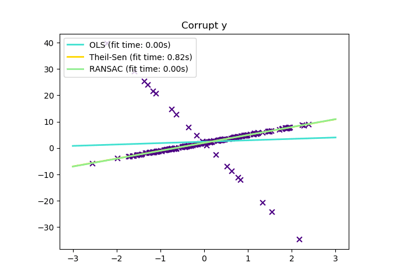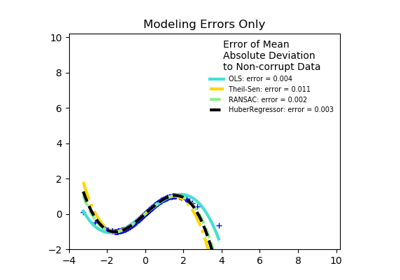/scikit-learn

# sklearn.linear_model.TheilSenRegressor

`class sklearn.linear_model.TheilSenRegressor(fit_intercept=True, copy_X=True, max_subpopulation=10000.0, n_subsamples=None, max_iter=300, tol=0.001, random_state=None, n_jobs=None, verbose=False)` [source]

Theil-Sen Estimator: robust multivariate regression model.

The algorithm calculates least square solutions on subsets with size n_subsamples of the samples in X. Any value of n_subsamples between the number of features and samples leads to an estimator with a compromise between robustness and efficiency. Since the number of least square solutions is “n_samples choose n_subsamples”, it can be extremely large and can therefore be limited with max_subpopulation. If this limit is reached, the subsets are chosen randomly. In a final step, the spatial median (or L1 median) is calculated of all least square solutions.

Read more in the User Guide.

Parameters: `fit_intercept : boolean, optional, default True` Whether to calculate the intercept for this model. If set to false, no intercept will be used in calculations. `copy_X : boolean, optional, default True` If True, X will be copied; else, it may be overwritten. `max_subpopulation : int, optional, default 1e4` Instead of computing with a set of cardinality ‘n choose k’, where n is the number of samples and k is the number of subsamples (at least number of features), consider only a stochastic subpopulation of a given maximal size if ‘n choose k’ is larger than max_subpopulation. For other than small problem sizes this parameter will determine memory usage and runtime if n_subsamples is not changed. `n_subsamples : int, optional, default None` Number of samples to calculate the parameters. This is at least the number of features (plus 1 if fit_intercept=True) and the number of samples as a maximum. A lower number leads to a higher breakdown point and a low efficiency while a high number leads to a low breakdown point and a high efficiency. If None, take the minimum number of subsamples leading to maximal robustness. If n_subsamples is set to n_samples, Theil-Sen is identical to least squares. `max_iter : int, optional, default 300` Maximum number of iterations for the calculation of spatial median. `tol : float, optional, default 1.e-3` Tolerance when calculating spatial median. `random_state : int, RandomState instance or None, optional, default None` A random number generator instance to define the state of the random permutations generator. If int, random_state is the seed used by the random number generator; If RandomState instance, random_state is the random number generator; If None, the random number generator is the RandomState instance used by `np.random`. `n_jobs : int or None, optional (default=None)` Number of CPUs to use during the cross validation. `None` means 1 unless in a `joblib.parallel_backend` context. `-1` means using all processors. See Glossary for more details. `verbose : boolean, optional, default False` Verbose mode when fitting the model. `coef_ : array, shape = (n_features)` Coefficients of the regression model (median of distribution). `intercept_ : float` Estimated intercept of regression model. `breakdown_ : float` Approximated breakdown point. `n_iter_ : int` Number of iterations needed for the spatial median. `n_subpopulation_ : int` Number of combinations taken into account from ‘n choose k’, where n is the number of samples and k is the number of subsamples.

#### Examples

```>>> from sklearn.linear_model import TheilSenRegressor
>>> from sklearn.datasets import make_regression
>>> X, y = make_regression(
...     n_samples=200, n_features=2, noise=4.0, random_state=0)
>>> reg = TheilSenRegressor(random_state=0).fit(X, y)
>>> reg.score(X, y)
0.9884...
>>> reg.predict(X[:1,])
array([-31.5871...])
```

#### Methods

 `fit`(X, y) Fit linear model. `get_params`([deep]) Get parameters for this estimator. `predict`(X) Predict using the linear model `score`(X, y[, sample_weight]) Returns the coefficient of determination R^2 of the prediction. `set_params`(**params) Set the parameters of this estimator.
`__init__(fit_intercept=True, copy_X=True, max_subpopulation=10000.0, n_subsamples=None, max_iter=300, tol=0.001, random_state=None, n_jobs=None, verbose=False)` [source]
`fit(X, y)` [source]

Fit linear model.

Parameters: `X : numpy array of shape [n_samples, n_features]` Training data `y : numpy array of shape [n_samples]` Target values `self : returns an instance of self.`
`get_params(deep=True)` [source]

Get parameters for this estimator.

Parameters: `deep : boolean, optional` If True, will return the parameters for this estimator and contained subobjects that are estimators. `params : mapping of string to any` Parameter names mapped to their values.
`predict(X)` [source]

Predict using the linear model

Parameters: `X : array_like or sparse matrix, shape (n_samples, n_features)` Samples. `C : array, shape (n_samples,)` Returns predicted values.
`score(X, y, sample_weight=None)` [source]

Returns the coefficient of determination R^2 of the prediction.

The coefficient R^2 is defined as (1 - u/v), where u is the residual sum of squares ((y_true - y_pred) ** 2).sum() and v is the total sum of squares ((y_true - y_true.mean()) ** 2).sum(). The best possible score is 1.0 and it can be negative (because the model can be arbitrarily worse). A constant model that always predicts the expected value of y, disregarding the input features, would get a R^2 score of 0.0.

Parameters: `X : array-like, shape = (n_samples, n_features)` Test samples. For some estimators this may be a precomputed kernel matrix instead, shape = (n_samples, n_samples_fitted], where n_samples_fitted is the number of samples used in the fitting for the estimator. `y : array-like, shape = (n_samples) or (n_samples, n_outputs)` True values for X. `sample_weight : array-like, shape = [n_samples], optional` Sample weights. `score : float` R^2 of self.predict(X) wrt. y.
`set_params(**params)` [source]

Set the parameters of this estimator.

The method works on simple estimators as well as on nested objects (such as pipelines). The latter have parameters of the form `<component>__<parameter>` so that it’s possible to update each component of a nested object.

Returns: self

## Examples using `sklearn.linear_model.TheilSenRegressor`Theil-Sen RegressionRobust linear estimator fitting